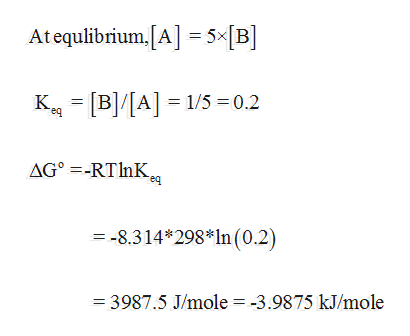# a. Which value corresponds to a negative value of ?G°: Keq = 10-2 or Keq = 102?b. In a unimolecular reaction with ve times as much starting material as product at equilibrium, what is the value of Keq? Is ?G° positive or negative?c. Which value corresponds to a larger Keq: ?G° = -8 kJ/mol or ?G° = 20 kJ/mol?

Question
4 views

a. Which value corresponds to a negative value of ?G°: Keq = 10-2 or Keq = 102?
b. In a unimolecular reaction with ve times as much starting material as product at equilibrium, what is the value of Keq? Is ?G° positive or negative?
c. Which value corresponds to a larger Keq: ?G° = -8 kJ/mol or ?G° = 20 kJ/mol?

check_circle

Step 1

a. Greater the equilibrium constant value the concentration of product formation is high.  An equilibrium reaction that favours product formation has negative standard gibbs free energy.  Therefore, Keq = 102 corresponds to negative standard Gibbs free energy.

Step 2help_outlineImage TranscriptioncloseAtequlibrium.[A] = 5×[B] K = [B]/[A] = 1/5 =0.2 AG° =-RTINK. req = -8.314*298*ln(0.2) = 3987.5 J/mole = -3.9875 kJ/mole fullscreen

### Want to see the full answer?

See Solution

#### Want to see this answer and more?

Solutions are written by subject experts who are available 24/7. Questions are typically answered within 1 hour.*

See Solution
*Response times may vary by subject and question.
Tagged in

### Chemistry